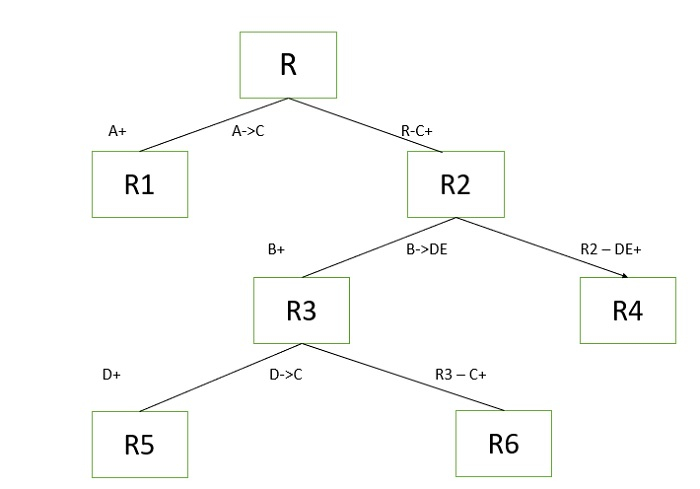# Check which FD violates 3NF in a given relation and decompose R into 3NF(DBMS)

A relation is in 3NF when it is in 2NF and there is no transitive dependency or a relation is in 3NF when it is in 2NF and all non-key attributes directly depend on candidate key.

Third normal form (3NF) is the third step in normalizing a database and it builds on the first and second normal forms, 1NF and 2NF.

3NF states that all column references in referenced data that are not dependent on the primary key should be removed. Another way of putting this is that only foreign key columns should be used to reference another table, and no other columns from the parent table should exist in the referenced table.

## Problem

For a given relation R(ABCDE) and F: {A->C, B->DE, D->C}, check which FD violates 3NF and decompose R into 3NF.

## Solution

Given F: {A->C, B->DE, D->C}

A+= AC => A is not candidate key

B+= BDEC => B is not candidate key

D+= DC => D is not candidate key

AB+=ACBDE => AB is candidate key

=> Key attribute =A,B and non-key attribute =C,D,E.

A->C violates 3NF [since A->C is transitive dependency]

B->DE violates 3NF [since B->DE is transitive dependency]

D->C violates 3NF [since D->C is transitive dependency].

3NF decomposition is as follows −We consider FDs which violates 3NF −

For A->C R(ABCDE0 decomposed to R1(AC) and R2(ABDE).

For B->DE R2(ABDE) decomposes to R3(BDEC) and R4(AB).

For D->C R3(BDEC) decomposes to R5(DC) and R6(BDE).

=>3NF decomposition of relation R is R1(AC) , R4(AB), R5(DC), R6(BDE).

Updated on: 06-Jul-2021

3K+ Views